## Part IV — Linear Equations

You have 30 minutes to complete this section of the Entrance Exam.

1.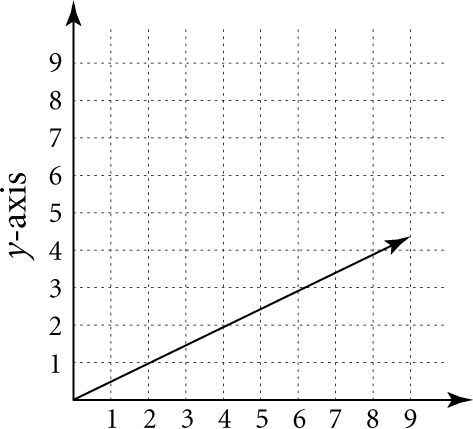According to the figure above,
A. as x increases, y decreases.
B. as x increases, y remains constant.
C. as x remains constant, y decreases.
D. as x decreases, y increases.
E. as x increases, y increases.
ABCDE

2.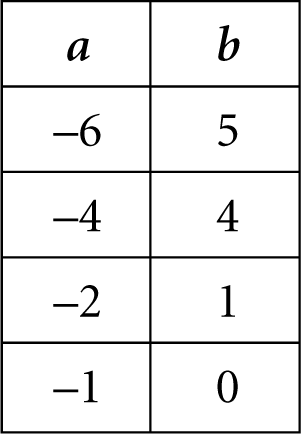Which of the following accurately describes the trends in the chart above?
A. As a increases, b decreases.
B. As a increases, b increases.
C. As a decreases, b decreases.
D. As a decreases, b increases.
E. As a decreases, b does not change.
ABCDE

3.Which statement is true for the given data?
A. As s increases, t decreases.
B. As s decreases, t does not change.
C. As s increases, t does not change.
D. As s increases, t increases.
E. As s decreases, t decreases.
ABCDE

#### For Questions 4–19, match the equation to the appropriate graph.

4. 3x – 5y = 29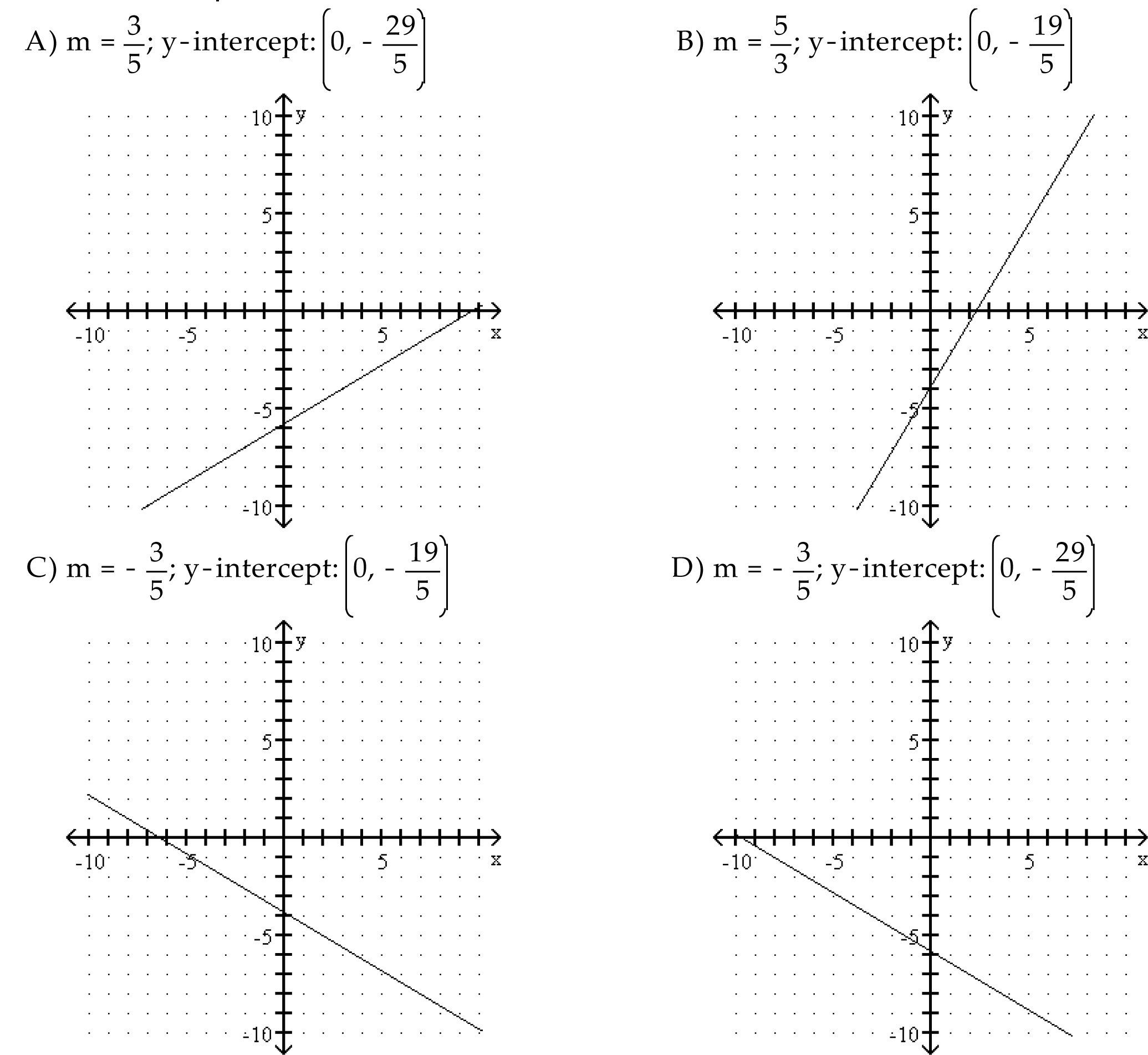ABCD

5. y = – ½ x – 5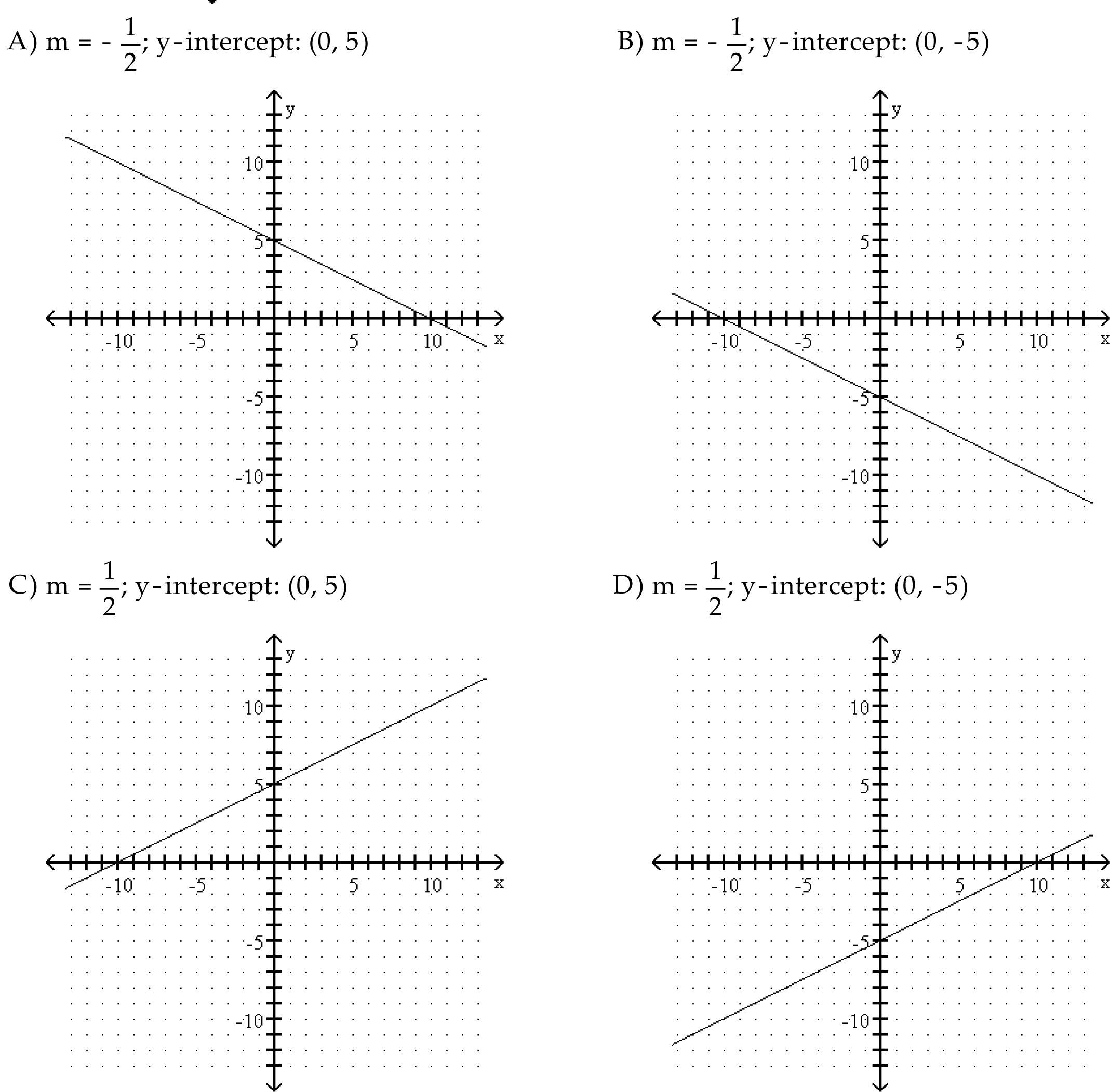ABCD

6. x + y = –3ABCD

7. 3x + 4y = 25ABCD

8. y = 2x – 5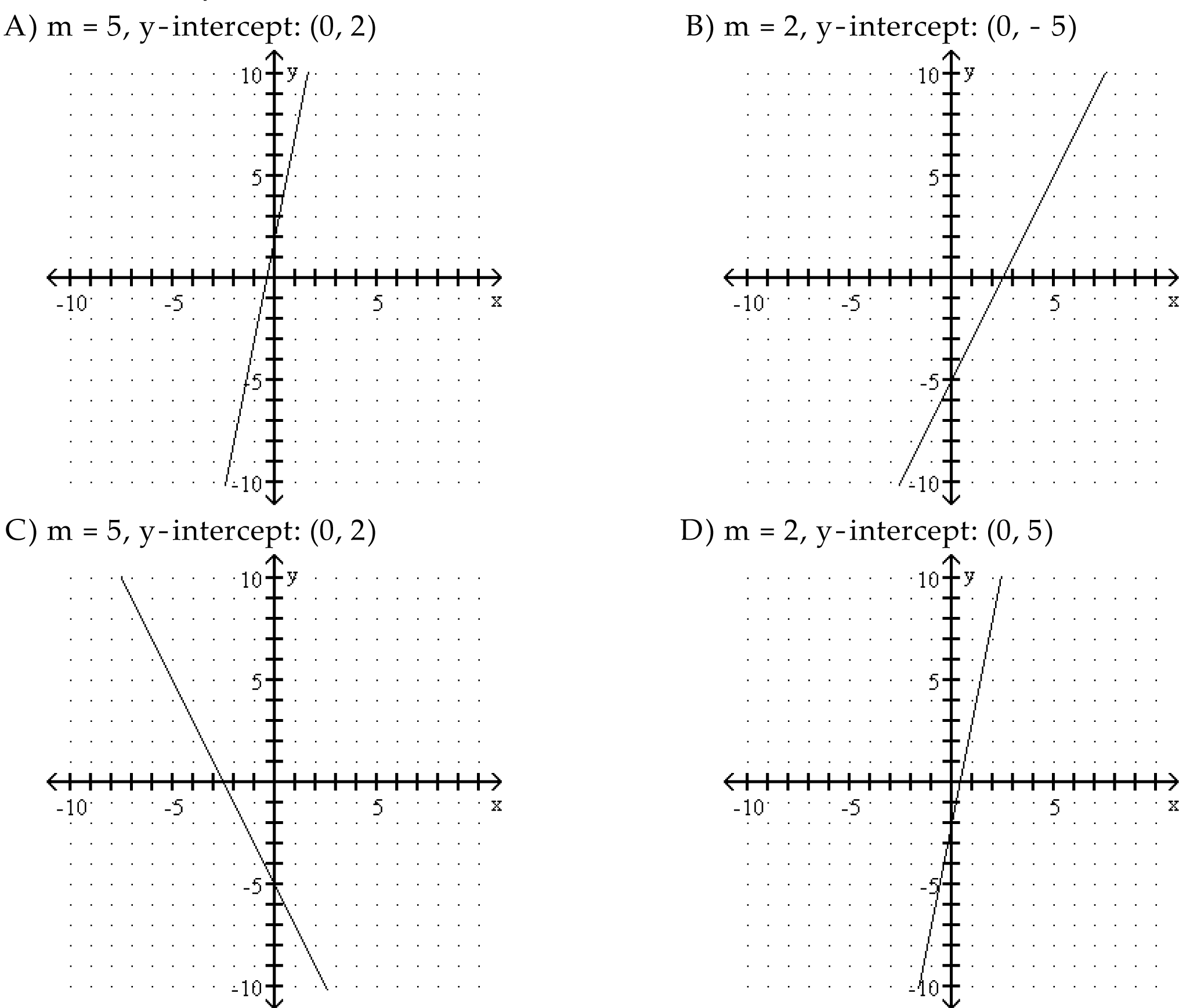ABCD

9. y = –4x + 5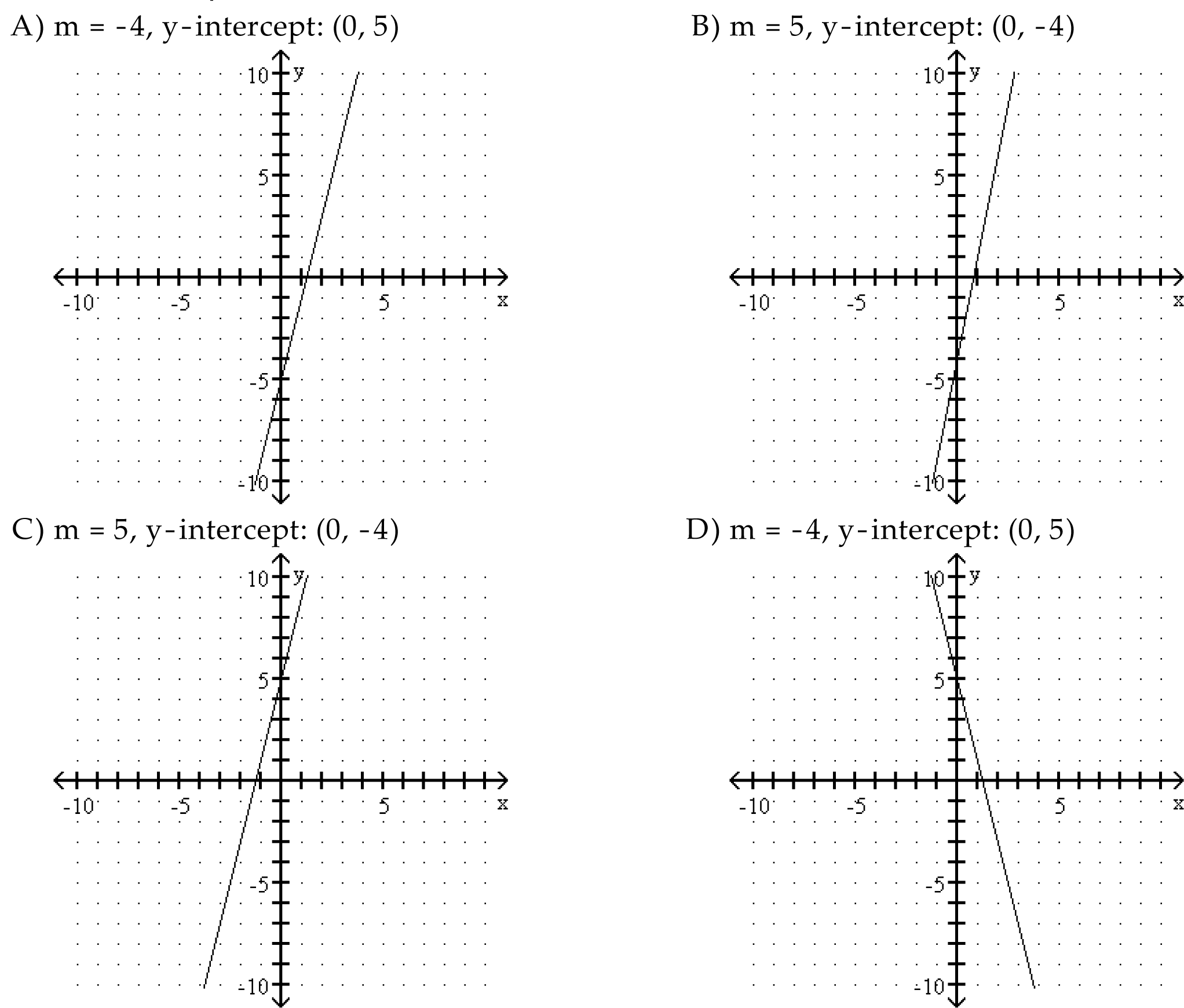ABCD

10. –5x + 2y + 7 = 0ABCD

11. –5y = –3x – 19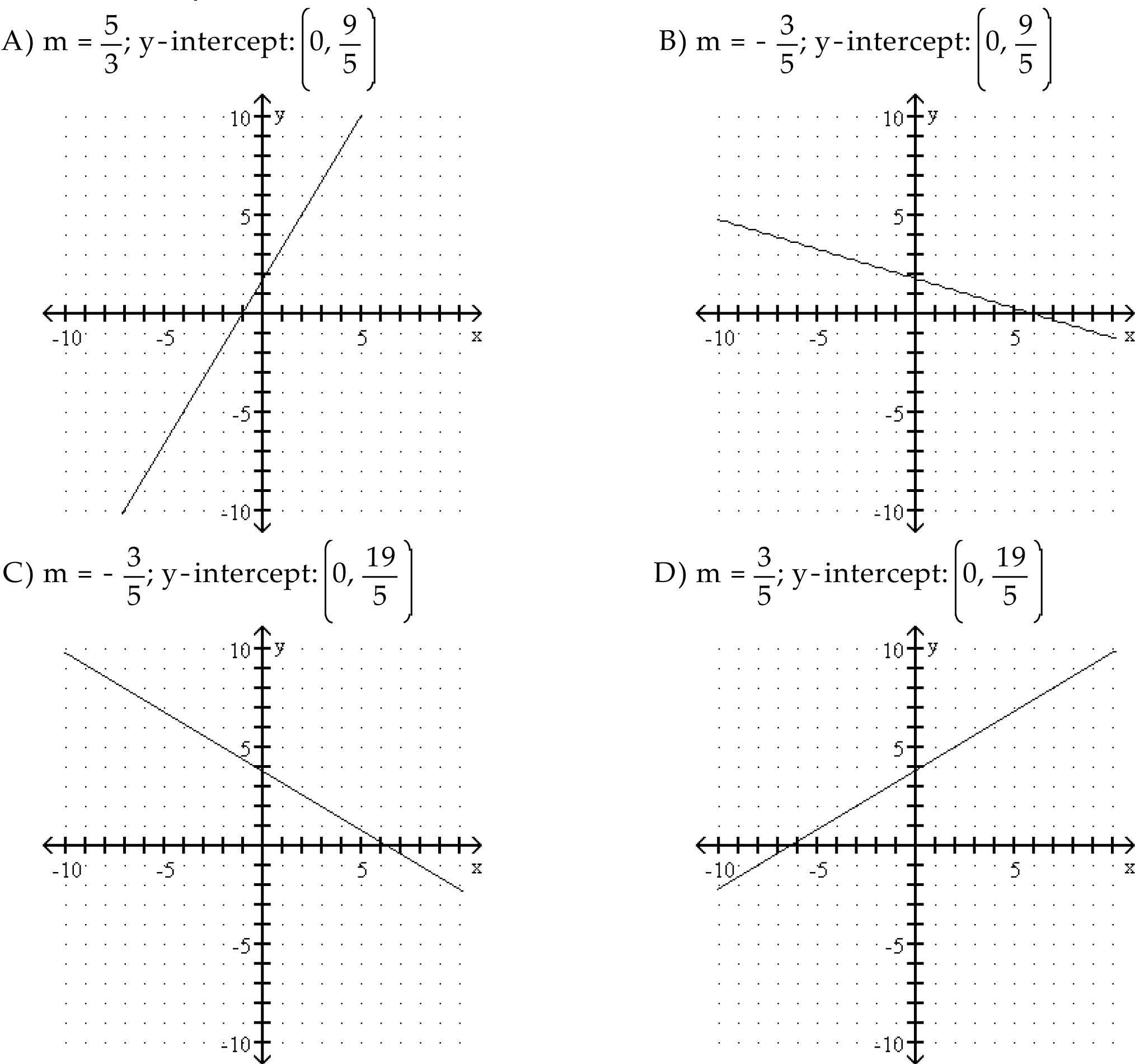ABCD

12. 4x – 5y = –36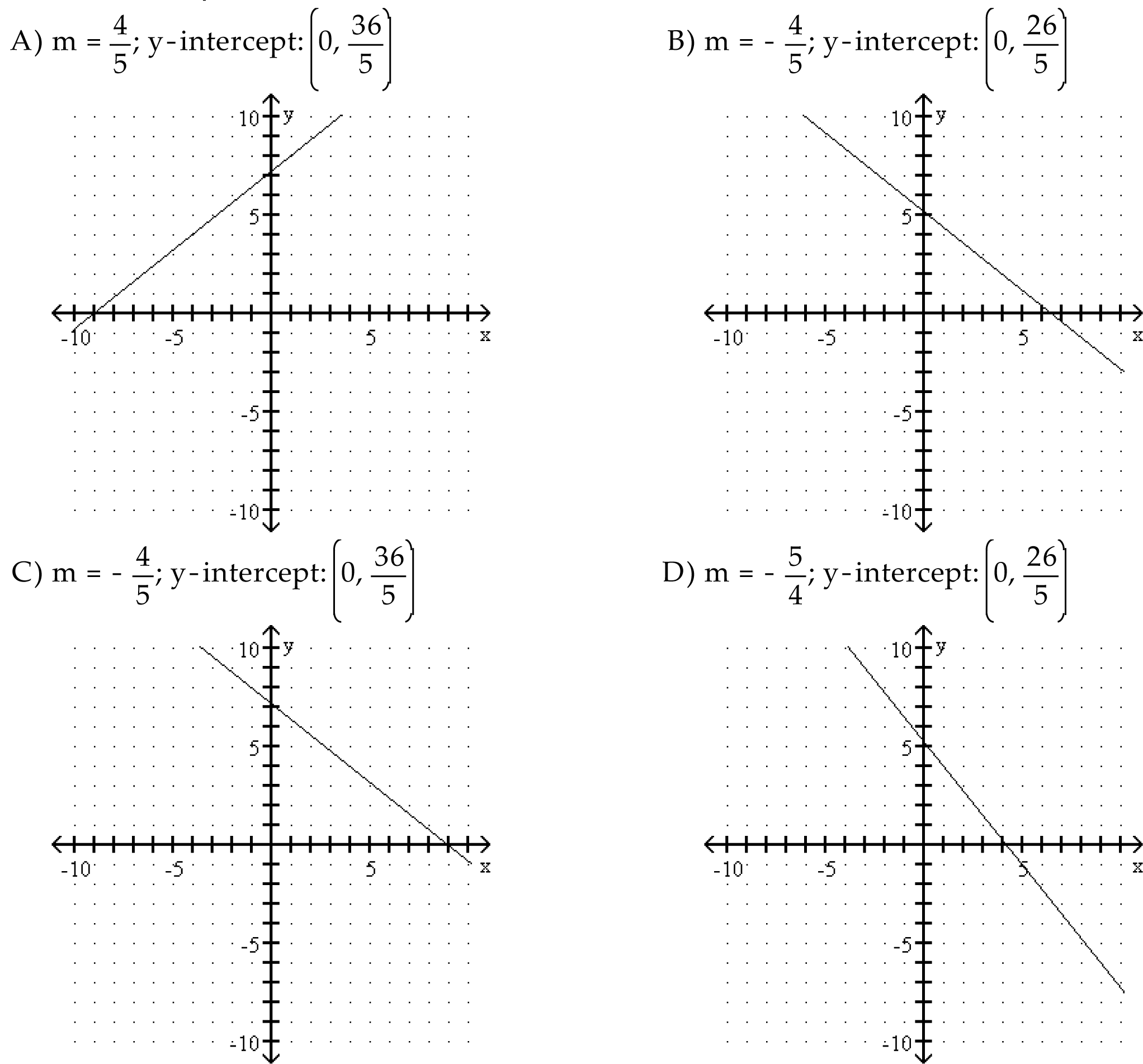ABCD

13. y = –2x – 5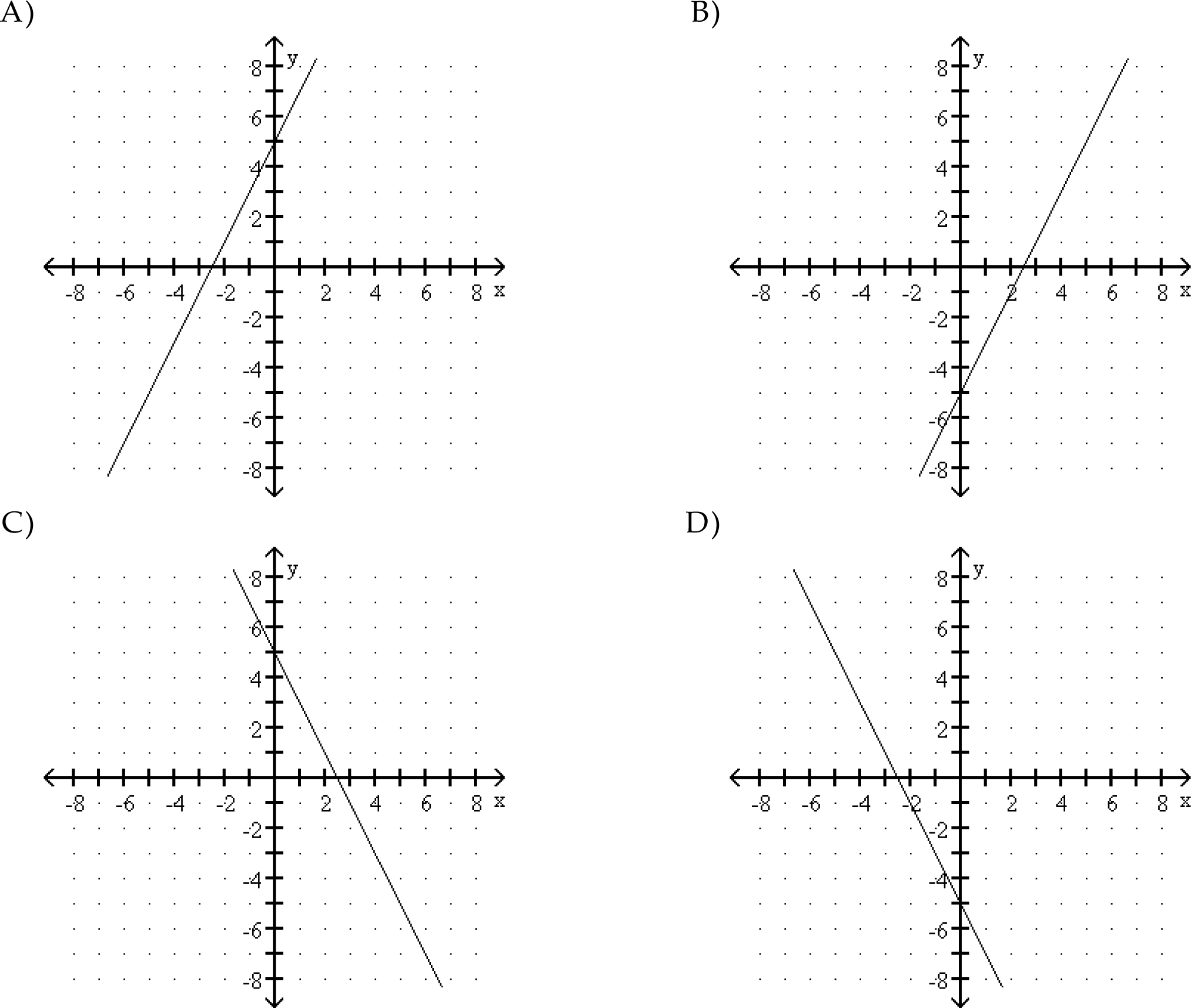ABCD

14. y = 5x + 6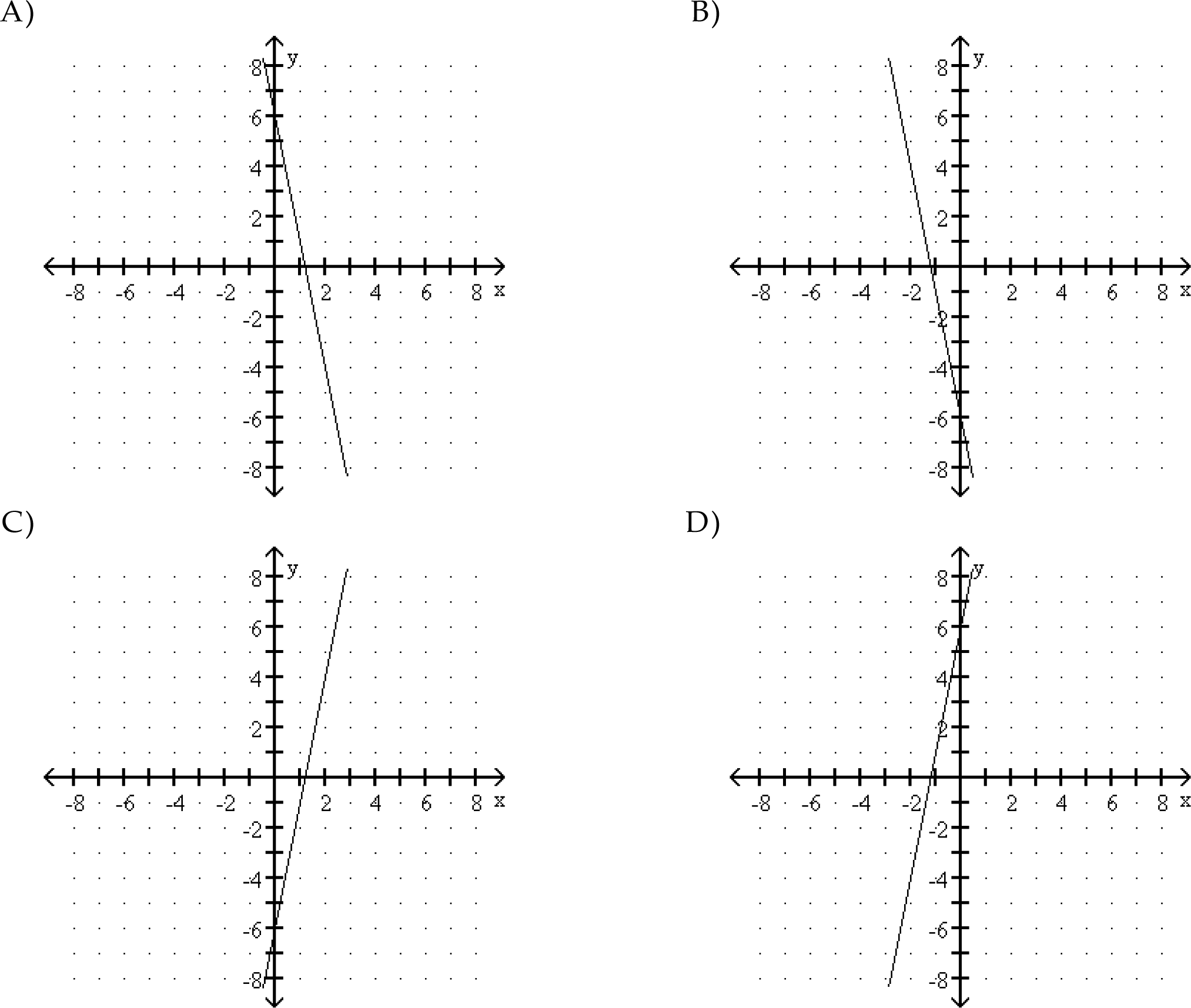ABCD

15. x = –y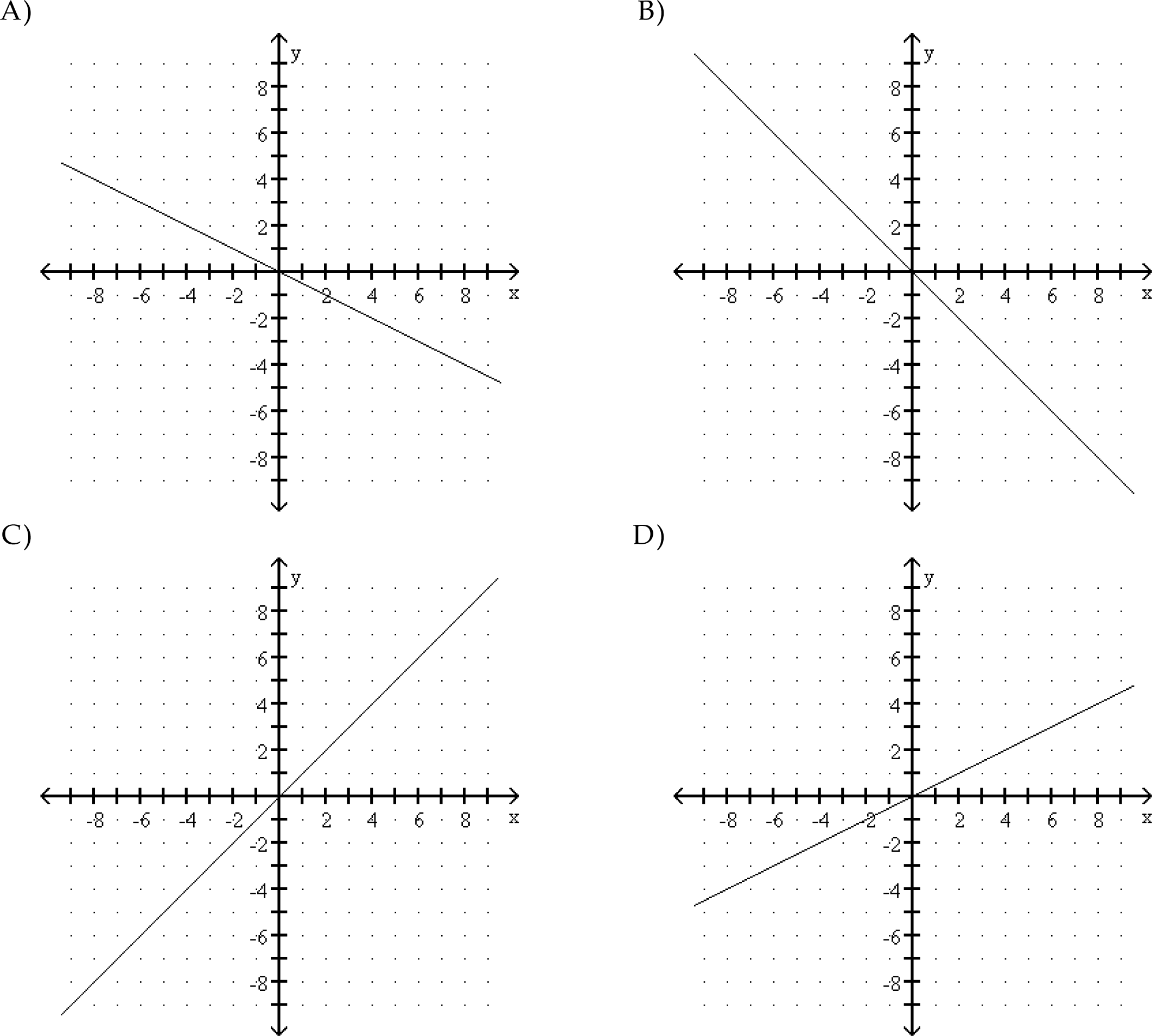ABCD

16. y = 5x – 4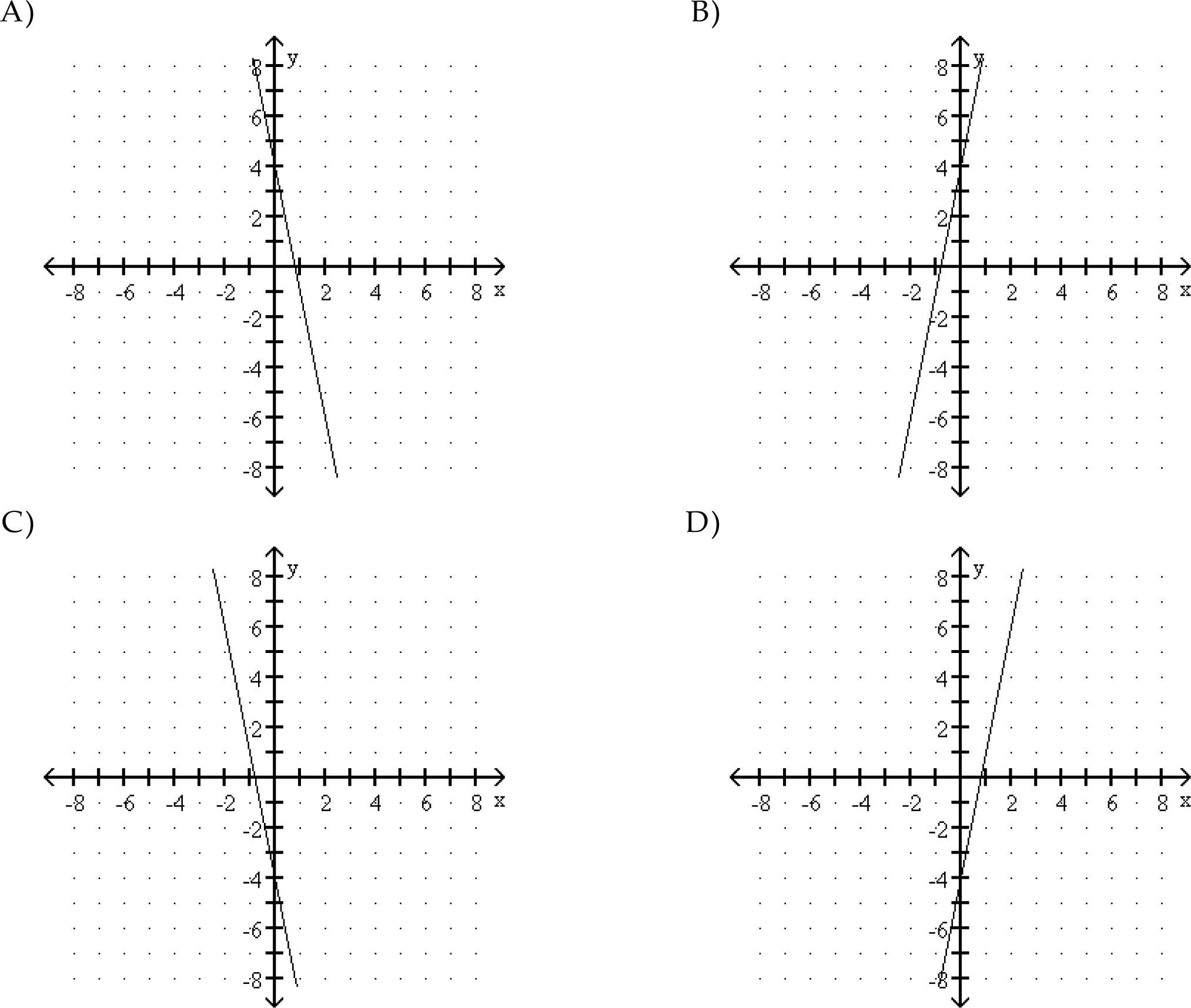ABCD

17. 2x – 4y = –9ABCD

18. y = –1ABCD

19. y = –5x + 4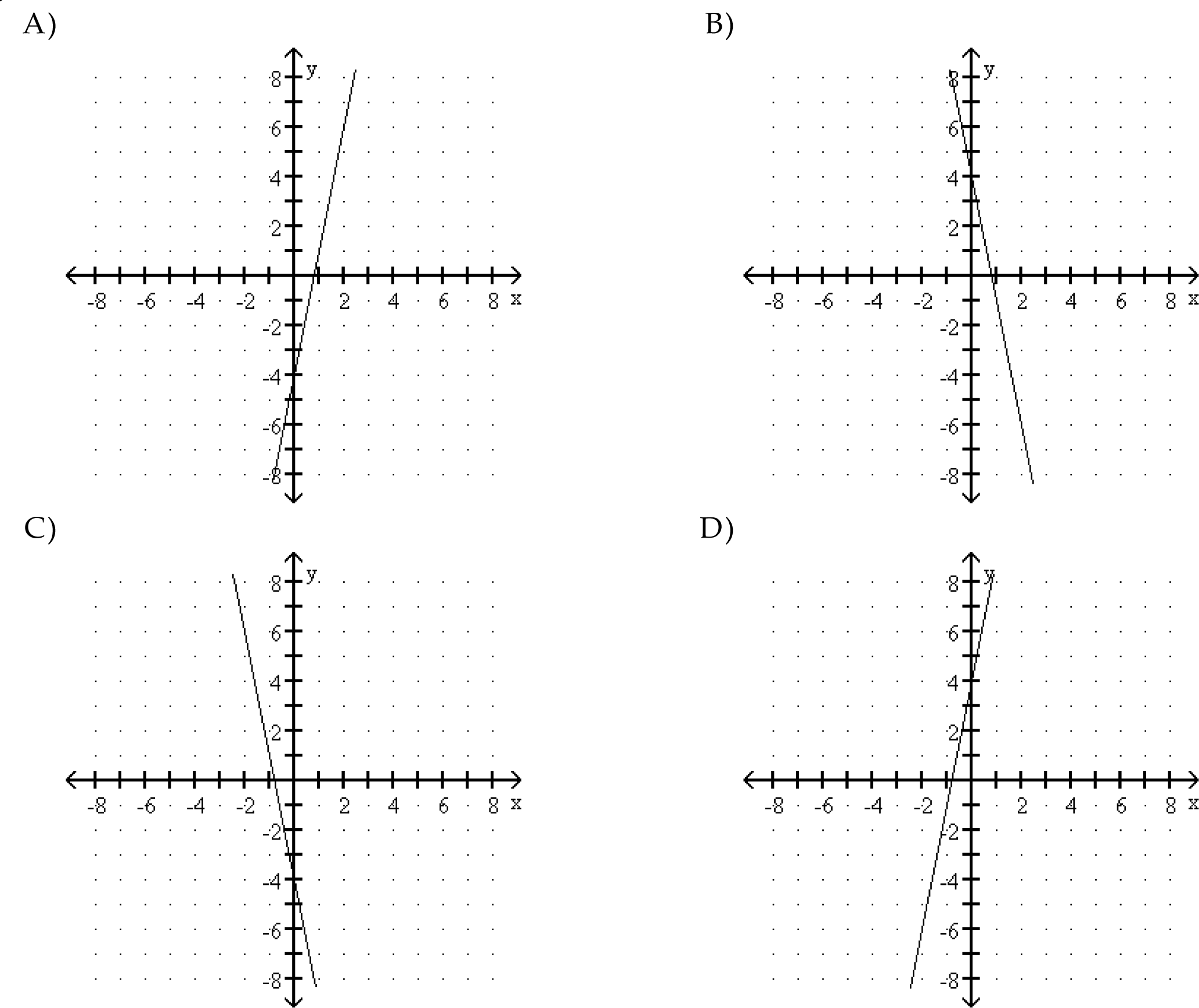ABCD

#### For questions 20–25, choose the correct equation of the graphed line in slope-intercept form.

NOTE: if a fraction precedes x, the x is NEVER in the denominator.

20.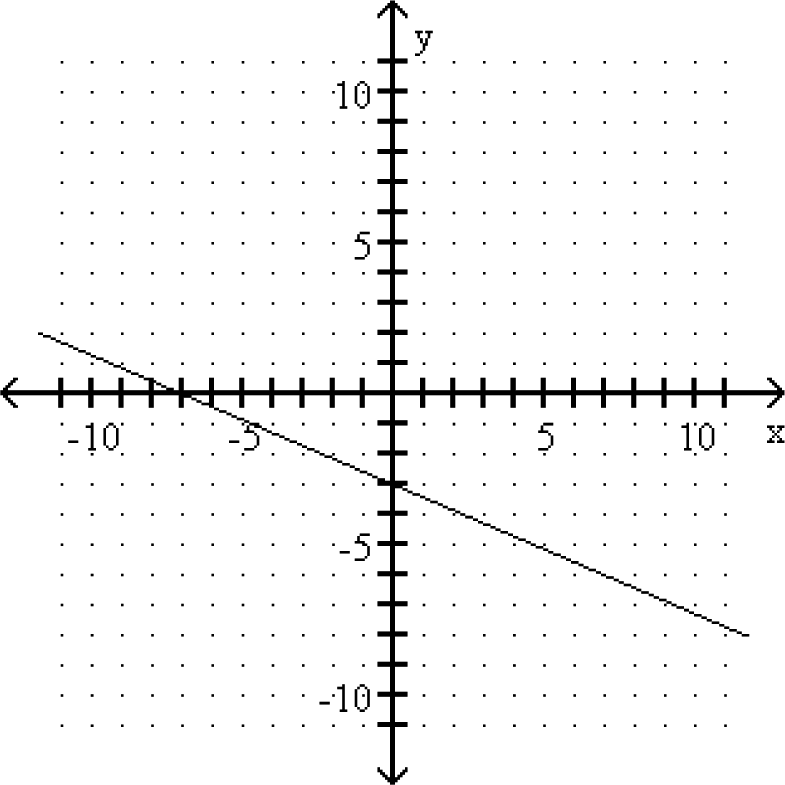A. y = −x − 7
B. y = −3/7 x − 3
C. y = −7/3 x − 7
D. y = −x − 3

ABCD

21.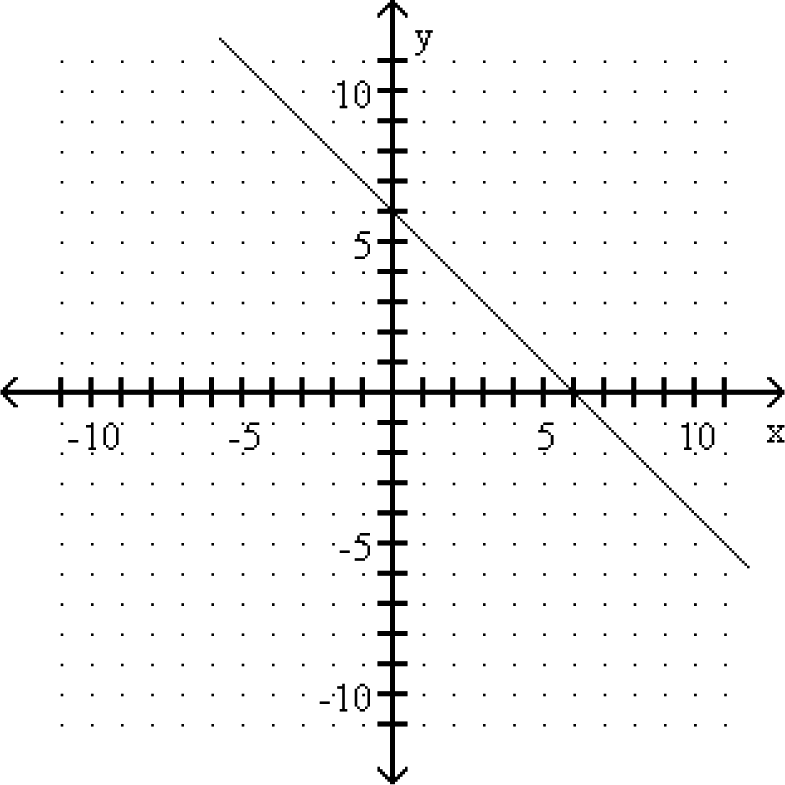A. y = x + 6
B. y = −x − 6
C. y = −x + 6
D. y = x − 6

ABCD

22.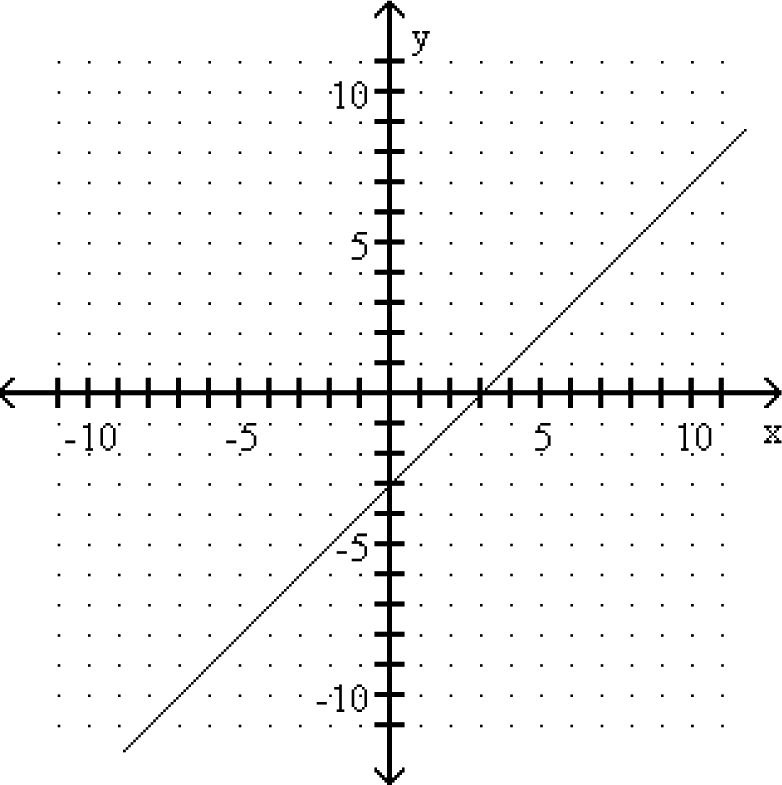A. y = x + 3
B. y = x − 3
C. y = −x − 3
D. y = −x + 3

ABCD

23.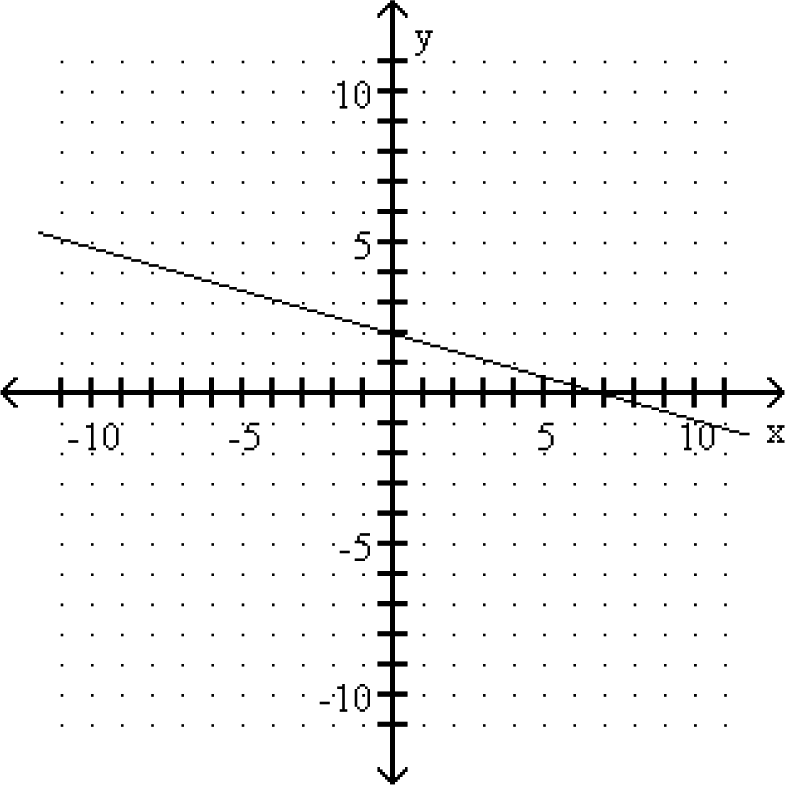A. y = 7x + 7
B. y = x + 2
C. y = −2/7 x + 2
D. y = −7/2 x + 7

ABCD

24.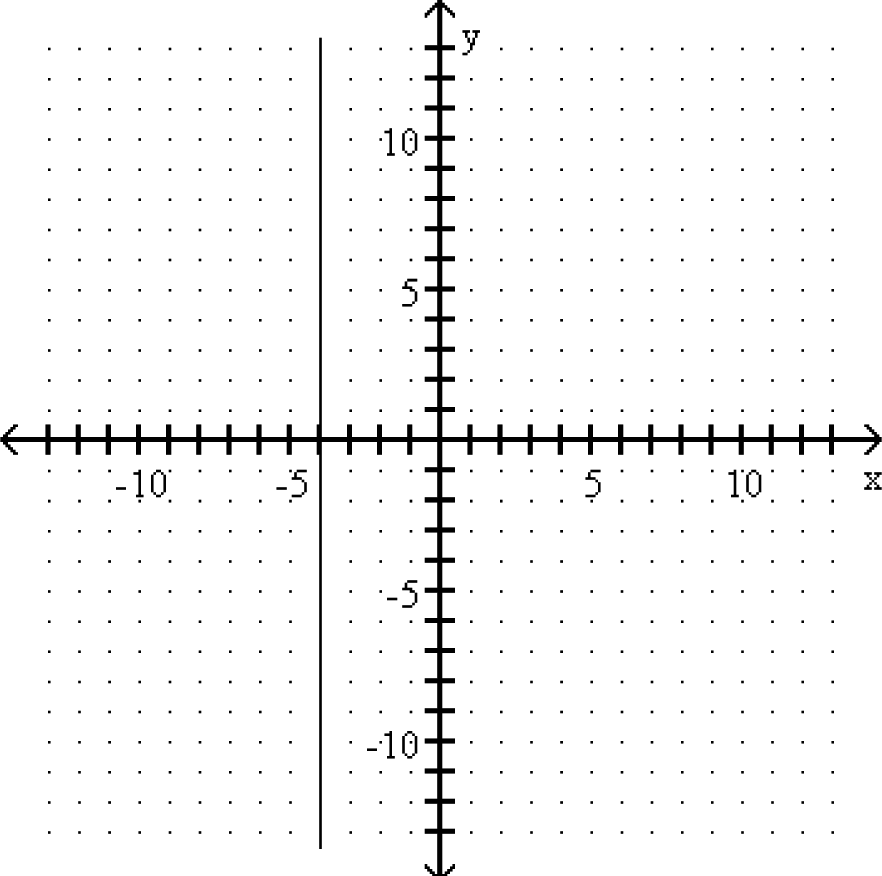A. y = −4
B. x = −4
C. y = 4
D. x = 4

ABCD

25.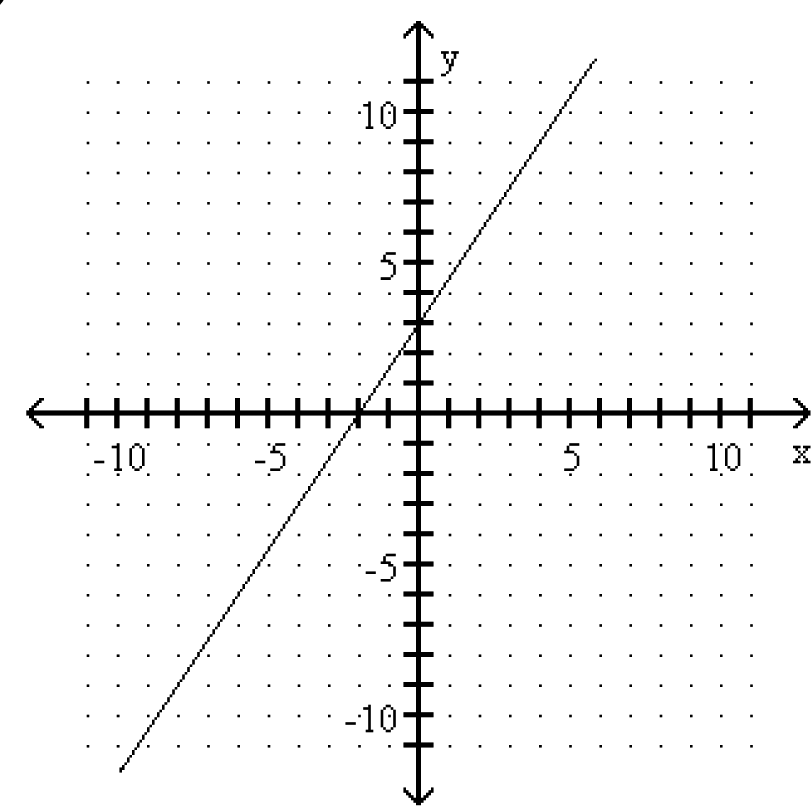A. y = 3/2 x + 3
B. y = x + 3
C. y = −2x − 2
D. y = 2/3 x − 2

ABCD